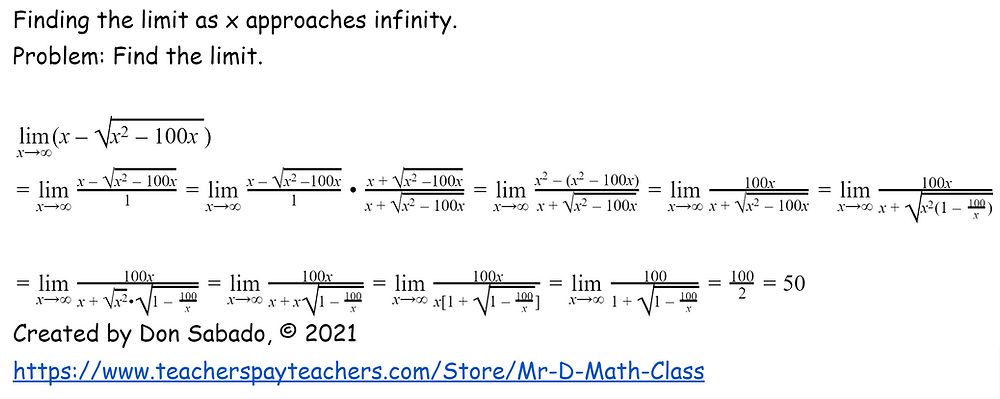Search

# Finding Limits as x approaches infinity

Sometimes it is not obvious when finding limits. In some situations, on the surface, the limit is unbounded and there is no limit. However, Using techniques learned in Precalculus, you can create a different form of the function where it becomes clear after multiplying the numerator and denominator by the conjugate of the numerator that the function has a limit. In this type of problem, make sure the Algebra process is clear and correct because we are rewriting the function in a different form using conjugates. The problem below is an example that illustrates the notion that on first inspection, the limit is not always as it seems at first thought. Rewriting the function by multiplying the numerator and denominator by the conjugate of the numerator and then factor out the x in the numerator creates a nice and neat answer as x approaches infinity.The one thing a student needs to worry about is that the student can get lost in the Algebra process and forget to place the limit in the correct location in the process. In the end the limit approaches 50 as x approaches infinity. This is definitely a bonus type of problem that I would give my AP Calculus AB students. To verify the limit, write the function into Desmos Graphing and you will see the graph approach 50 as x approaches infinity.